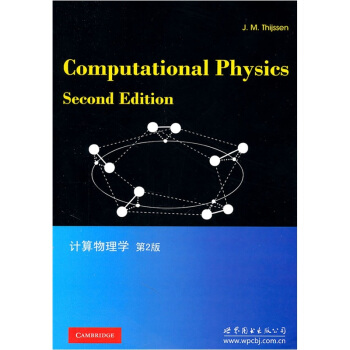# 计算物理学（第2版） [Computational Physics Second Edition] 下载 mobi epub pdf

[荷] 蒂森 著

## 图书介绍

ISBN：9787510032905

## 图书描述

### 内容简介

《计算物理学（第2版）》是一部理论物理研究的计算方法的教程。这是第二版，在第一版的基础上做了大量的更新，内容更加全面。新增加的部分包括，有限元方法，格点boltzmann模拟，密度函数理论，量子分子动力学，monte carlo模拟和一维量子系统的对角化。书中囊括了了物理研究的很多不同方面和不同计算方法论。如monte carlo方法和分子模拟动力学以及各种电子结构方法论，偏微分方程解方法，格点规范理论。全书都在强调不同物理场中的方法之间的关系，内容较为简洁明快，具有基本编程，数值分析，场论以及凝聚态理论和统计物理的本科知识背景就可以完全读懂《计算物理学（第2版）》。不管是理论物理，计算物理还是实验物理专业的研究生还是科研人员，《计算物理学（第2版）》都相当有参考价值。目次：导论；具有球对称势的量子散射；schrdinger方程的变分大法；hartree-fock方法；密度函数理论；周期性固态schr.dinger方程解法；经典平衡态统计力学；分子动力学模拟；量子分子动力学；monte carlo方法；变换矩阵和自旋链的对角化；量子monte carlo方法，偏微分方程的有限元方法，流体力学的lattice boltzmann方法，格点场论的计算方法；高效能计算和并行法；附：数值法；随机数发生器。

### 目录

preface to the first edition
preface to the second edition
1 introduction
1.1 physics and computational physics
1.2 classical mechanics and statistical mechanics
1.3 stochastic simulations
1.4 electrodynamics and hydrodynamics
1.5 quantum mechanics
1.6 relations between quantum mechanics and classical statistical physics
1.7 quantum molecular dynamics
1.8 quantum field theory
exercises
references
2 quantum scattering with a spherically symmetric
potential
2.1 introduction
2.2 a program for calculating cross sections
2.3 calculation of scattering cross sections

exercises
references
3 the variational method for the schr'odinger equation
3.1 variational calculus
3.2 examples of variational calculations
3.3 solution of the generalised eigenvalue problem
3.4 perturbation theory and variational calculus
exercises
references
4 the hartree-fock method
4.1 introduction
4.2 the bom-oppenheimer approximation and the independent-particle method
4.3 the helium atom
4.4 many-electron systems and the slater determinant
4.5 self-consistency and exchange: hartree-fock theory
4.6 basis functions
4.7 the structure of a hartree-fock computer program
4.8 integrals involving gaussian functions
4.9 applications and results
4.10 improving upon the hartree-fock approximation
exercises
references
5 density functional theory
5.1 introduction
5.2 the local density approximation
5.3 exchange and correlation: a closer look
5.4 beyond dft: one- and two-particle excitations
5.5 a density functional program for the helium atom
5.6 applications and results
exercises
references
6 solving the schriodinger equation in periodic solids
6.1 introduction: definitions
6.2 band structures and bloch's theorem
6.3 approximations
6.4 band structure methods and basis functions
6.5 augmented plane wave'methods
6.6 the linearised apw （lapw） method
6.7 the pseudopotential method
6.8 extracting information from band structures
6.10 other band methods
exercises
references
7 classical equilibrium statistical mechanics
7.1 basic theory
7.2 examples of statistical models; phase transitions
7.3 phase transitions
7.4 determination of averages in simulations
exercises
references
molecular dynamics simulations
8.1 introduction
8.2 molecular dynamics at constant energy
8.3 a molecular dynamics simulation program for argon
8.4 integration methods: symplectic integrators
8.5 molecular dynamics methods for different ensembles
8.6 molecular systems
8.7 long-range interactions
8.8 langevin dynamics simulation
8.9 dynamical quantities: nonequilibrium molecular dynamics
exercises
references
9 quantum molecular dynamics
9.1 introduction
9.2 the molecular dynamics method
9.3 an example: quantum molecular dynamics for the hydrogen molecule
9.4 orthonormalisation; conjugate gradient and rm-diis techniques
9.5 implementation of the car-parrinello technique for pseudopotential dft
exercises
references
10 the monte carlo method
10.1 introduction
10.2 monte carlo integration
10.3 importance sampling through markov chains
10.4 other ensembles
10.5 estimation of free energy and chemical potential
10.6 further applications and monte carlo methods
10.7 the temperature of a finite system
exercises
references
11 transfer matrix and diagonalisation of spin chains
11.1 introduction
11.2 the one-dimensional ising model and the transfer matrix
11.3 two-dimensional spin models
11.4 more complicated models
11.5 'exact' diagonalisation of quantum chains
11.6 quantum renormalisation in real space
11.7 the density matrix renormalisation group method
exercises
references
12 quantum monte carlo methods
12.1 introduction
12.2 the variational monte carlo method
12.3 diffusion monte carlo
12.4 path-integral monte carlo
12.5 quantum monte carlo on a lattice
12.6 the monte carlo transfer matrix method
exercises
references
13 the finite element method for partial differential equations
13.1 introduction
13.2 the poisson equation
13.3 linear elasticity
13.4 error estimators
13.5 local refinement
13.6 dynamical finite element method
13.7 concurrent coupling of length scales: fem and md
exercises
references
14 the lattice boltzmann method for fluid dynamics
14.1 introduction
14.2 derivation of the navier-stokes equations
14.3 the lattice boltzmann model
14.5 derivation of the navier-stokes equation from the
lattice boltzmann model
exercises
references
15 computational methods for lattice field theories
15.1 introduction
15.2 quantum field theory
15.3 interacting fields and renormalisation
15.4 algorithms for lattice field theories
15.5 reducing critical slowing down
15.6 comparison of algorithms for scalar field theory
15.7 gauge field theories
exercises
references
16 high performance computing and parallelism
16.1 introduction
16.2 pipelining
16.3 parallelism
16.4 parallel algorithms for molecular dynamics
references
appendix a numerical methods
a2 iterative procedures for special functions
a3 finding the root of a function
a4 finding the optimum of a function
a5 discretisation
a7 differential equations
a8 linear algebra problems
a9 the fast fourier transform
exercises
references
appendix b random number generators
b1 random numbers and pseudo-random numbers
b2 random number generators and properties of pseudo-random numbers
b3 nonuniform random number generators
exercises
references
index

### 精彩书摘

Now we can define the problems in a more abstract way. It is convenient toconsider continuum problems. The candidate solutions （for example the possibleconformations） form a phase space, and the merit function has some complicatedshape on that space - it contains many valleys and mountains, which can be verysteep. The solution we seek corresponds to the lowest valley in the landscape. Notethat the landscape is high-dimensional. You may think, naively, that a standardnumerical minimum finder can solve this problem for you. However, this is notthe case as such an algorithm always needs a starting point, from which it findsthe nearest local minimum, which is not necessarily the best you can find in theconformation space. The set of points which would go to one particular local min-imum when fed into a steepest descent or other minimum-finder （see AppendixA4） is called the basin of attraction of that minimum. Once we are in the basinof attraction of the global minimum we can easily find this global minimum; theproblem is to find its basin of attraction.
……

## 用户评价